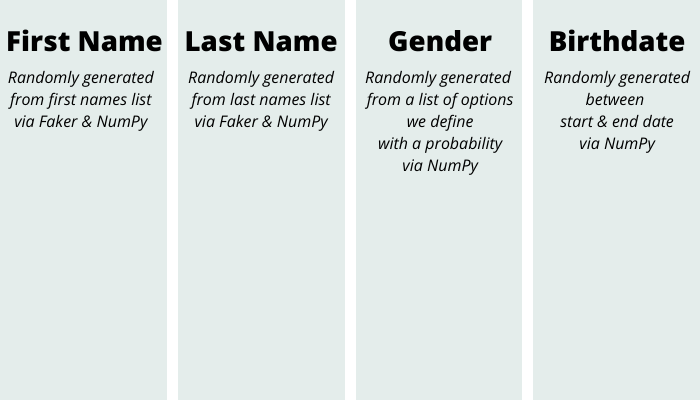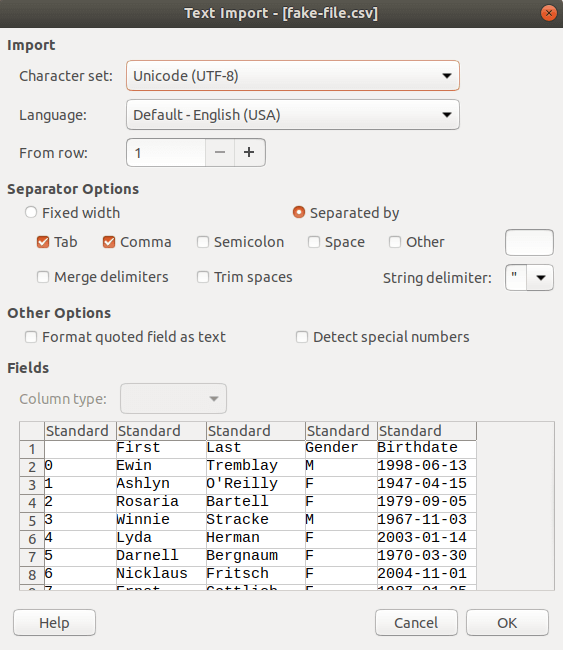Last August, our CTO Colin Copeland wrote about how to import multiple Excel files in your Django project using pandas. We have used pandas on multiple Python-based projects at Caktus and are adopting it more widely.

Since Colin’s post, pandas released version 1.0 in January of this year and is currently up to version 1.0.3. Pandas is fairly popular in the data analysis community. It was showcased at PyData NYC 2019, and was planned to be highlighted during multiple sessions at Pycon 2020 (before the event was canceled). A pandas core developer will give a keynote at the postponed PyData Miami 2020 event (date to be determined).

In this article, I’m going to take you through the steps to create some sample fake data in a CSV file. Large fake datasets can be useful when load testing your code. Pandas makes writing and reading either CSV or Excel files straight-forward and elegant.

## Using NumPy and Faker to Generate our Data

When we’re all done, we’re going to have a sample CSV file that contains data for four columns:We’re going to generate numPy ndarrays of first names, last names, genders, and birthdates. Once we have our data in ndarrays, we save all of the ndarrays to a pandas DataFrame and create a CSV file.

The ndarray data we’re generating in the next few methods will look a little like this:

```['Ann', 'Joe', 'Donna', 'Ansel', ...]
['Patel', 'Cho', 'Smith', 'García', ...]
['F', 'M', 'M', 'O', ...]  # Female, Male, Other, and Blank are our gender options in this example
['1961-01-16', '1982-03-01', '1995-09-12', '1987-08-07', ...]
```

### Let's Get Started!

First, let’s import pandas, NumPy, and some Faker providers. We are using NumPy and Faker to randomly generate fake data.

```import numpy as np
import pandas as pd
from faker.providers.person.en import Provider
```

Next, let’s create some functions to randomly generate our data for names,

```def random_names(name_type, size):
"""
Generate n-length ndarray of person names.
name_type: a string, either first_names or last_names
"""
names = getattr(Provider, name_type)
return np.random.choice(names, size=size)
```

for gender,

```def random_genders(size, p=None):
"""Generate n-length ndarray of genders."""
if not p:
# default probabilities
p = (0.49, 0.49, 0.01, 0.01)
gender = ("M", "F", "O", "")
return np.random.choice(gender, size=size, p=p)
```

and for birthdate.

```def random_dates(start, end, size):
"""
Generate random dates within range between start and end.
"""
# Unix timestamp is in nanoseconds by default, so divide it by
# 24*60*60*10**9 to convert to days.
divide_by = 24 * 60 * 60 * 10**9
start_u = start.value // divide_by
end_u = end.value // divide_by
return pd.to_datetime(np.random.randint(start_u, end_u, size), unit="D")
```

Now, let’s call each of these methods and generate a sample CSV file using a pandas DataFrame.

```# How many records do we want to create in our CSV? In this example
# we are generating 100, but you could also find relatively fast results generating
# much larger datasets
size = 100
df = pd.DataFrame(columns=['First', 'Last', 'Gender', 'Birthdate'])
df['First'] = random_names('first_names', size)
df['Last'] = random_names('last_names', size)
df['Gender'] = random_genders(size)
df['Birthdate'] = random_dates(start=pd.to_datetime('1940-01-01'), end=pd.to_datetime('2008-01-01'), size=size)

df.to_csv('fake-file.csv')
```

That’s it! Now we have a fake file with records of people we generated with pandas, NumPy, and Faker in milliseconds.Opening the CSV file we generated

### Pandas Proves to be Efficient and Effective

As you can see, pandas makes readable and succinct code for writing directly to our CSV columns by header name. We found that this combination of pandas, Faker lists, and NumPy methods makes generating fake sample data fast and efficient.